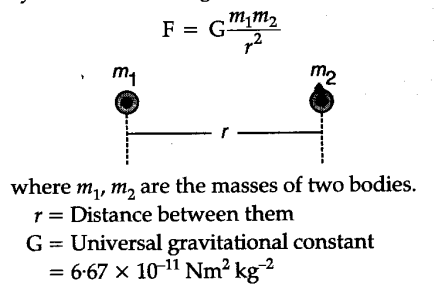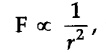# Write short note on fundamental forces in nature

There are following four fundamental forces in nature:
(i) Gravitational forces, (ii) electromagnetic forces, (iii) strong forces or nuclear forces, (iv) weak forces. Some of the important features of these forces are discussed below:
(i) Gravitational forces : These are the forces of attraction between any two bodies in the universe separated by a definite distance. These are governed by Newton’s law of gravitation:Characteristics of gravitational forces :
(i) They are always attractive. They are never repulsive. They exist between microscopic as well as macroscopic bodies.
(ii) They are the weakest forces in nature.
(iii) They are central forces in nature, i.e., they set along the line joining the centres of two bodies.
(iv) They are conservative forces.
(v) They obey inverse square law, i.e.,they vary inversely as the square of the
distance between the two bodies.
(vi) They are long range forces, i.e., gravitational forces between any two bodies exist even when their distance of separation is quite large.
(vii) The field particle of gravitational forces is called
gravitors. The concept of exchange of field particles between two bodies explains how the two bodies interact from a distance.
(ii) Electromagnetic forces : They include the electrostatic and magnetic forces. The electrostatic forces are the forces between two static charges while magnetic forces are the forces between two
magnetic poles. The moving charges give rise to the magnetic forces. The combined action of these forces are called electromagnetic forces. Characteristics of electromagnetic forces :
(i) These forces are both attractive as well as repulsive.
(ii) They are central forces in nature.
(iii) They obey inverse square law.
(iv) They are conservative forces in nature.
(v) These forces are due to the exchange of particles known as photons which carry no charge and have zero rest mass.
(vi) They are 1036 times stronger as compared to
gravitational forces and 10u times stronger than the weak forces.
(iii) Strong forces : The particles inside the nucleus are charged particles (protons) and neutral particles (neutrons) which are bounded to each other by a strong interaction called nuclear force or strong force. Hence, they may be defined as the forces binding the nucleons (protons and neutrons) together in a nucleus. These are responsible for the stability of the atomic nucleus. These are of three types:
(i) n-n forces are the forces of attraction between two neutrons.
(ii) p-p forces are the forces of attraction between two protons.
(iii) n-p forces are the forces of the attraction between a proton and a neutron.
Characteristics of nuclear forces:
(i) They are basically attractive in nature and become repulsive when the distance between nucleons is less than 0.5 Fermi.
(ii) They obey inverse square law.
(iv) Weak forces : They are defined as the interactions which take place between elementary particles during radioactive decay of a radioactive substance. In p-decay the nucleus emits an electron and a particle called neutrino (which is uncharged). The interaction between the electron and the neutrino is known as weak interaction or weak forces. Characteristics of weak forces:
(i) These are the types of forces that we encounter in macroscopic world, while.
(ii) These are the types of forces that we encounter in
the microscopic world.